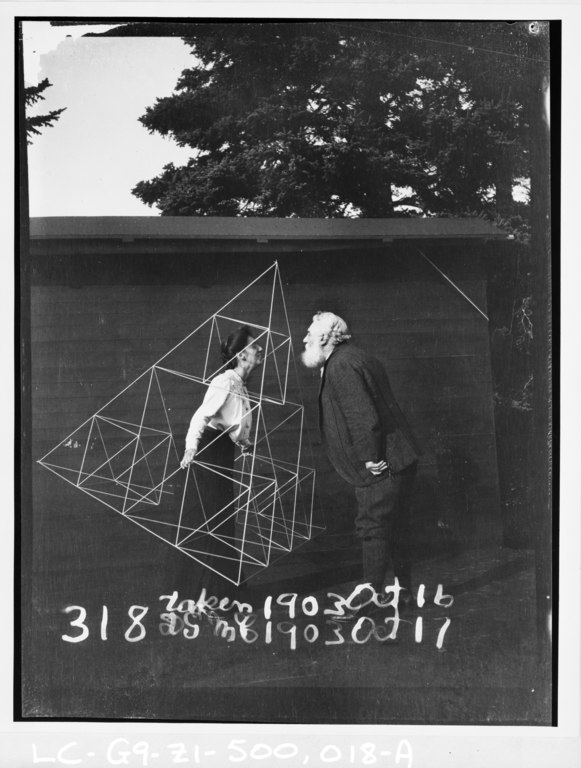The Hanoi graphs and Sierpiński graphs both look like the Sierpiński triangle, and have a very similar recursive construction from triples of smaller graphs of the same type, but they are not quite the same graphs as each other. The Sierpiński graphs (left, below) are the graphs of the vertices and boundary edges of partially-constructed Sierpiński triangles; they can also be formed from three smaller Sierpiński graphs by identifying pairs of extreme vertices (the vertices of degree two at the three corners of the triangular layout). The Hanoi graphs (right, below) are the state spaces of the tower of Hanoi puzzle, in which rings of different size are moved one at a time between three pegs, only allowing moves that keep the rings sorted on each peg. They also have a construction from three smaller Hanoi graphs, but where the pairs of extreme vertices are connected by an edge rather than identified.The difference between them comes out much more strongly when you generalize them to higher dimensions. The Sierpiński triangle generalizes to tetrahedra (a popular shape for kites) and higher-dimensional simplices; the photo below shows Mabel Bell and Alexander Graham Bell, seemingly about to kiss, in a three-dimensional Sierpiński graph, the framework for a kite.Again, the $$d$$-dimensional Sierpiński graph has a recursive construction from $$d+1$$ smaller graphs of the same type, identified at extreme vertices (the vertices of degree $$d$$ at the $$d+1$$ corners of the layout). Because the number of vertices separating the subgraphs at each level of the recursion is so small, these graphs have bounded treewidth, and a few years ago on the TCS stackexchange I calculated the treewidth of the Sierpiński triangle graphs explicitly as being four. The same bound transfers easily enough to the three-peg Hanoi graphs.

The analogue of higher dimensions for the Hanoi graphs is to use more pegs. The Hanoi graph with $$p$$ pegs and $$r$$ rings has $$p^r$$ states, more or less the same as the Sierpiński graph for $$(d-1)$$-dimensional Sierpiński fractals with $$r$$ levels of recursion. Here’s the one with two rings; each state is described by a pair of letters, using a capital letter for the peg holding the larger ring and a lowercase letter for the peg holding the smaller ring.The recursive construction for these graphs combines $$p$$ copies of a smaller graph of the same type: one copy for each position where the largest ring can be placed, and a smaller graph describing the placements of the smaller rings once the largest ring has been placed. These copies of the smaller graph are connected together by edges describing the movements of the largest ring. But I’ve only drawn an example for two rings because these graphs get messy and hard to draw very quickly. The reason is not the exponential number of total vertices, but the large number of connections from one recursive subgraph to another. Two recursive subgraphs are connected whenever the largest ring can move from its peg in one subgraph to its peg in the other, and this is allowed whenever these two pegs have no smaller rings on them. So in a Hanoi graph with $$p$$ pegs, $$r$$ rings, and $$p^r$$ vertices, each pair of recursive subgraphs has $$(p-2)^{r-1}$$ edges between them, one for each placement of the smaller rings on the $$p-2$$ remaining pegs.

The recursive subdivision with $$(p-2)^{r-1}$$ edges between subgraphs leads to a tree-decomposition with treewidth $$O\bigl((p-2)^r\bigr)$$, and this naturally raises the question of whether this is tight or whether some other less-intuitive recursive decomposition has smaller cuts between its recursive subgraphs. This is the question studied in my newest preprint, “On the treewidth of Hanoi graphs” (arXiv:2005.00179), with UCI student Daniel Frishberg and Oregon State student Will Maxwell, to appear at FUN 2020 (supposedly to be held in person in September in Italy after being rescheduled from June, but I’m not holding my breath). We don’t get a precise answer, but we succeed in proving bounds on the treewidth of the form $$\Omega\bigl((p-2)^r/r^{O(1)}\bigr)$$. This is nearly tight for fixed $$p$$ and variable $$r$$: we get the same exponential function of $$r$$ as the upper bound, and are smaller than the upper bound by only a much lower-order polynomial factor. But the exact treewidth remains elusive.

To put it in a possibly more familiar form, when one of these graphs (for a fixed number of pegs and variable number of rings) has $$n$$ vertices, it has separators of size $$O(n^c)$$, where $$c=\log_p (p-2)$$. For the four-peg Hanoi graphs, this means separators of size $$O(\sqrt{n})$$, more or less the same as for planar graphs (although these graphs seem far from planar). But that nice exponent is just a coincidence caused by the fact that $$p=4$$ is a power of $$p-2=2$$. For other choices of $$p$$, that doesn’t happen and we get a transcendental exponent $$c$$. So these graphs don’t even act like $$(p-1)$$-dimensional graphs, for which a reasonable separator exponent might be the rational number $$(p-2)/(p-1)$$. And they certainly don’t act like the Sierpiński graphs, for which the exponent is zero.